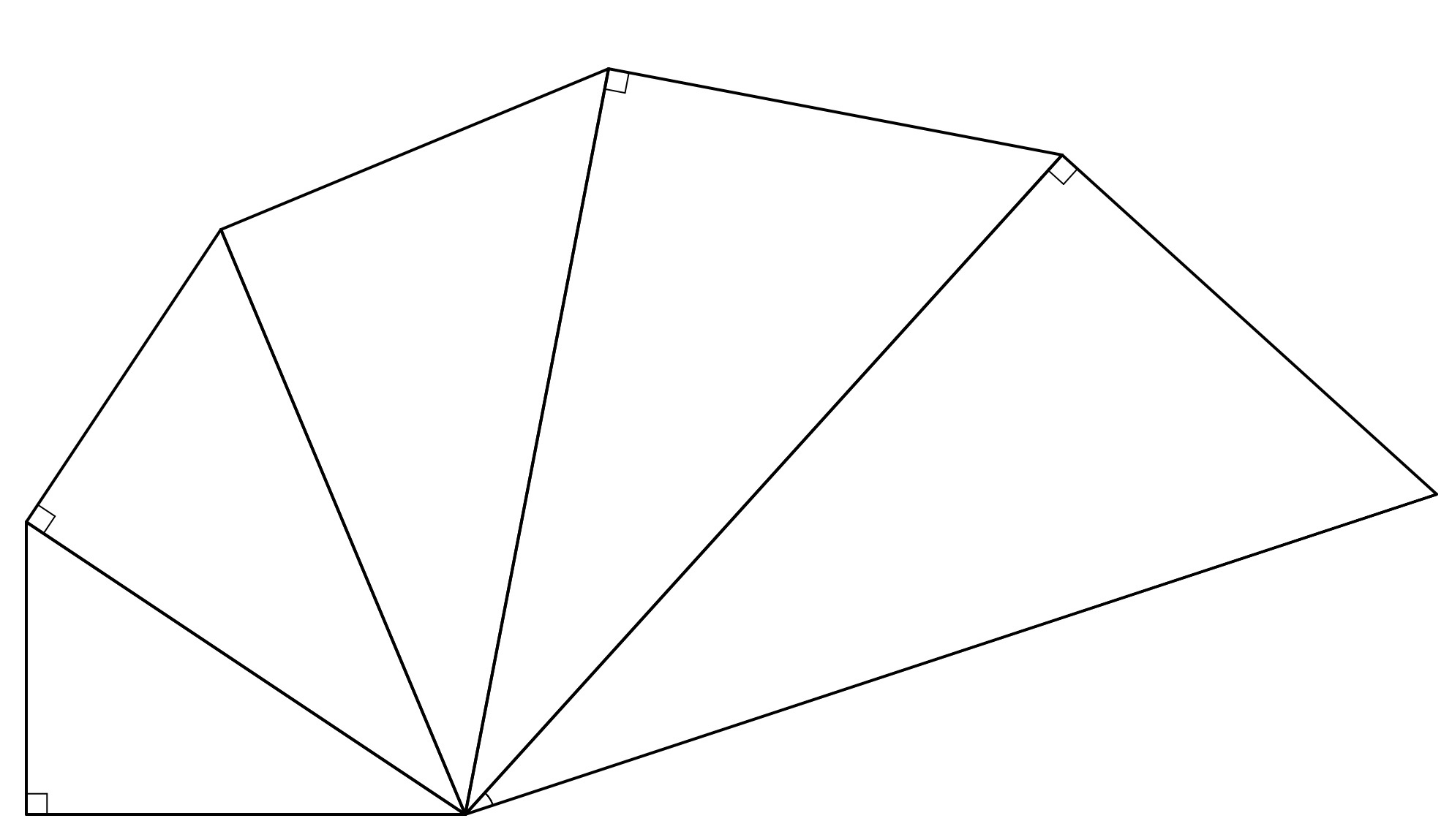Triangle spiral

Geometry Level 3A spiral of similar triangles like the one depicted above is constructed such that the smallest triangle (the one with the least perimeter) has a base of 4 and height of 3.

If the length of the 9th triangle's hypotenuse can be represented as $\frac{a}{b}$ for positive co-prime integers a,b, find a+b.

Use of a calculator is expected.

×